Computational & Technology Resources
an online resource for computational,
engineering & technology publications
Civil-Comp Proceedings
ISSN 1759-3433
CCP: 84
PROCEEDINGS OF THE FIFTH INTERNATIONAL CONFERENCE ON ENGINEERING COMPUTATIONAL TECHNOLOGY
Edited by: B.H.V. Topping, G. Montero and R. Montenegro
Paper 9

Generalised Fractional Differential Equations using 'Proper' Primitives

P.W. Krempl

AVL List GmbH, Graz, Austria

Full Bibliographic Reference for this paper
P.W. Krempl, "Generalised Fractional Differential Equations using 'Proper' Primitives", in B.H.V. Topping, G. Montero, R. Montenegro, (Editors), "Proceedings of the Fifth International Conference on Engineering Computational Technology", Civil-Comp Press, Stirlingshire, UK, Paper 9, 2006. doi:10.4203/ccp.84.9
Keywords: fractional calculus, primitives, integral transforms, Taylor series, fractional differential equations.

Summary
The concept of 'proper' primitives is presented. These functions are generalised complex derivatives satisfying the following rules:
1. The demand for coincidence with classical derivatives for integer real orders,
2. the rule of recursion combined with classical derivations or indefinite integration,
3. the rule of composition,
4. the rule of commutation (hence they form an Abelian group with respect to the derivatives),
5. they are continuous functions with respect to the order of the derivatives.

These 'proper' primitives are uniquely defined as families of functions where no assumptions about initial conditions are implied by the limits of integration, like in the case of the frequently used Riemann-Liouville definitions of fractional derivatives. These generalised complex derivatives can be calculated without any restriction to finite or infinite range of definition. They can therefore match any initial conditions imposed by the nature of the problem under investigation. These proper primitives as generalised complex derivatives can be generated via a transformation recently proposed  in an axiomatic way. Some of them are listed below: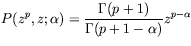with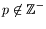(10)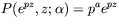with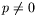(11)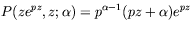with(12)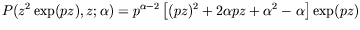with(13)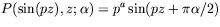with(14)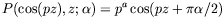with(15)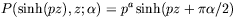with(16)with(17)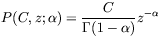(18)

Due to the non-vanishing derivatives of a constant, except for non-negative integer order, these derivatives are non-degenerated linear operators. The difference between the Taylor series of a function in classical calculus according to this concept is discussed.

These proper primitives can be used to solve linear differential equations containing derivatives of arbitrary real or even complex order. Linear differential equations of time independent systems can be solved by a similar concept as in the conventional case. If the solution of the characteristic equation consists of n roots, the general solution of the homogeneous differential equation contains a set of n proper primitives, and their n coefficients are determined by n initial conditions. The difference to the classical case, where a differential equation of the order n has usually to match n initial conditions for the function and its derivatives up to the order n-1, the choice of the orders of the derivatives subject to initial conditions is arbitrary from the mathematical point of view. This allows the selection of such initial conditions which are imposed within the context of the given problem as real observable quantities, like position and velocity of a moving body under a fractional damping law obeying a fractional differential equation. Simple examples are given as demonstration in the paper.

This concept of generalised complex derivatives allows the application of the theorems of function theory to the proper primitives as meromorphic functions of the order of the derivative as their complex variable. This yields insights into the mathematical nature of generalised derivatives, and is both complementary to the other, and very beneficial approaches to fractional calculus.

References
1
P.W. Krempl, "On the 'Proper' Primitives in Fractional Calculus". Proc. 2nd IFAC Workshop on Fractional Differentiation and its Applications (FDA'06), Porto, July 2006 (to be published)

purchase the full-text of this paper (price £20)